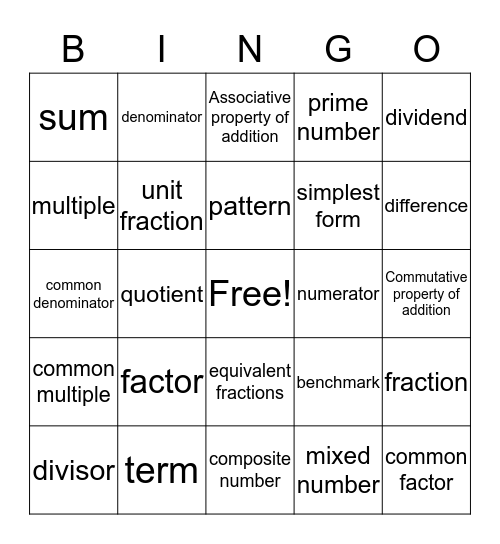# Math Vocab. BingoThis bingo card has a free space and 24 words: factor, term, pattern, divisor, dividend, sum, multiple, denominator, numerator, equivalent fractions, simplest form, common denominator, benchmark, fraction, unit fraction, mixed number, Commutative property of addition, Associative property of addition, difference, quotient, common factor, common multiple, prime number and composite number.

## Play Online